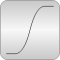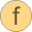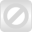# Special

Library of special mathematical functions# Package Contentserf Error function erf(u) = 2/sqrt(pi)*Integral_0_u exp(-t^2)*derfc Complementary error function erfc(u) = 1 - erf(u)erfInv Inverse error function: u = erf(erfInv(u))erfcInv Inverse complementary error function: u = erfc(erfcInv(u))sinc Unnormalized sinc function: sinc(u) = sin(u)/uInternal Internal utility functions that should not be directly utilized by the user

# Information

This information is part of the Modelica Standard Library maintained by the Modelica Association.

This sublibrary contains functions to compute often used mathematical operators that cannot be expressed analytically.